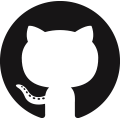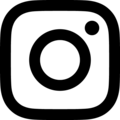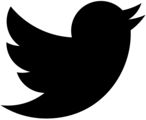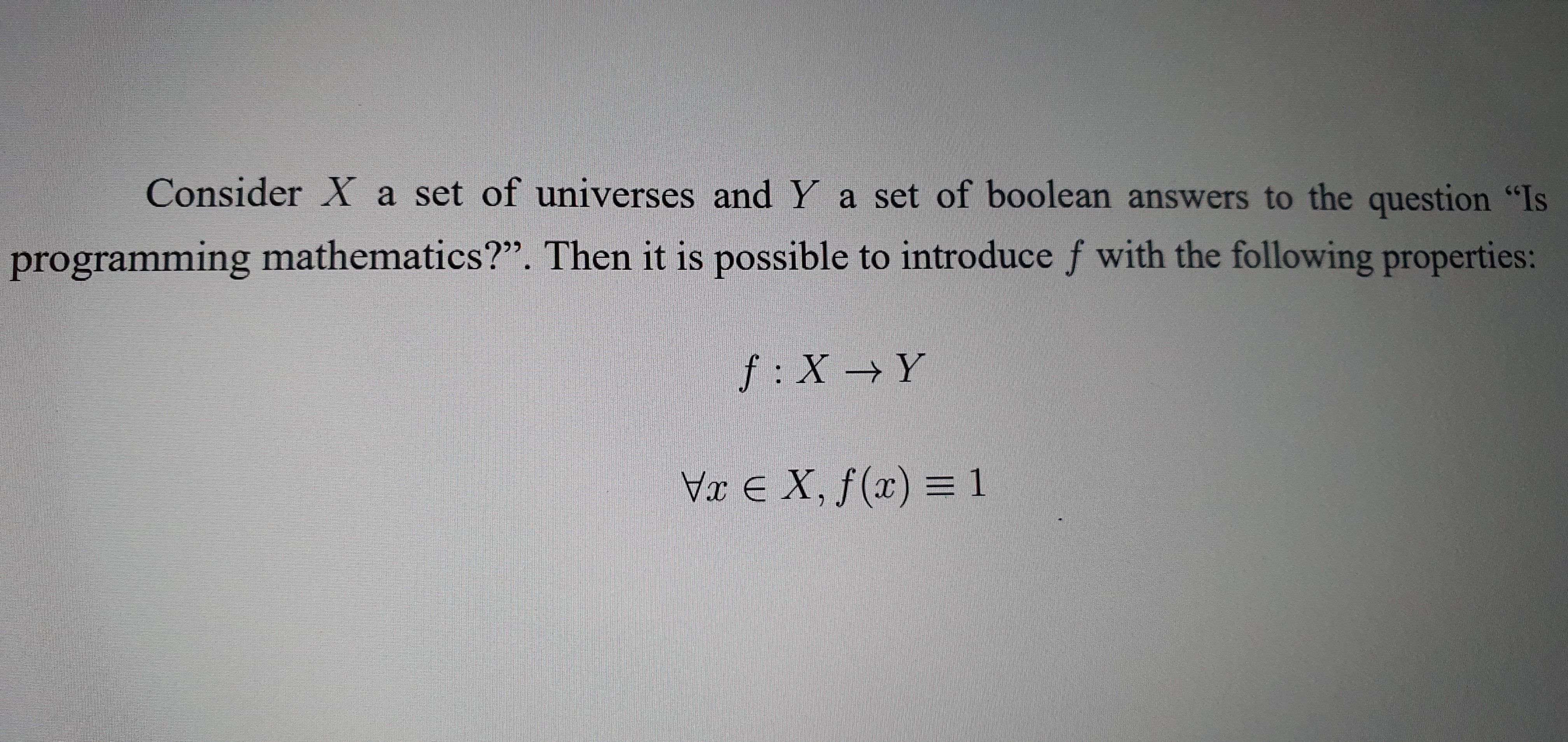Maxim MenshikovStatic analysis researcher and software engineer

What is mathematics?First of all, it is a science about abstract objects and their relations. Numbers are one sort of these abstract objects. Usually, numbers have no boundaries, so it is possible to represent ((-\infty, \infty)). The discrete mathematics adds some limitations to numbers, say, numbers can only be integral (it is a very “rough” definition: actually, discrete mathematics is about discreteness as opposed to continuity, but most people will only consider the part about “integral” vs “any” numbers). Then, there are lattices: partially ordered sets with infimums and supremums.

Numbers are not the only abstract objects, but surely among the most important ones. The presence of abstract objects is an important property of mathematics. Now, how are the numbers represented?

Point 1: types.

How are programming languages different from mathematics? In almost every language the user deals with strongly typed numbers that can have minimum and maximum value. Say, 8-bit integer has range ([0, 255]). To sum up two such integers, there must be an overflow rule which maps the sum to the ([0, 255]) range. So, types allow making mathematical objects having specific constraints. In many ways, it is similar to lattices, and it is an essential part of mathematics.

Point 2: the abstractness of expressions.

Why should (x = 5) be mathematical when written on a paper and not when written in C program? Why is (z = f(x, y)), (x,y \in [0, 255]), (f(x, y) = (x + y) \bmod 256) mathematical, but (z = x + y) is not? I would argue that programming language term is even more abstract, because it builds upon the axiomatic knowledge of types and their relations.

Point 3: programs are not “instructions for computers” when executed not by the computer they were built for.

As one may say, programming languages allow creating programs, essentially scripts for computers, so these programs are not mathematics, they are instructions for computers to execute. The latter is true for imperative languages as long as you execute them. Once you analyze the program, it is no longer instruction, it is a purely mathematical object.

Languages such as Coq make it possible to prove real-world theorems. Z3 and CVC4 languages deal with a significant part of math mechanics and can be used for proving statements. There are also declarative languages. Programs written in these languages don’t have a single instruction for the computer.

Conclusion, but there are a lot more arguments.

These days programming languages represent modern mathematical notation. It lacks a lot of “magic” of pure Descartes notation, but it was a huge effort to unite mathematics and engineering. It is not only boolean logic. Everything that can be written mathematically can be written in code. The language mightn’t have all features needed to represent the special notations, but it is up to the user to choose the right language.